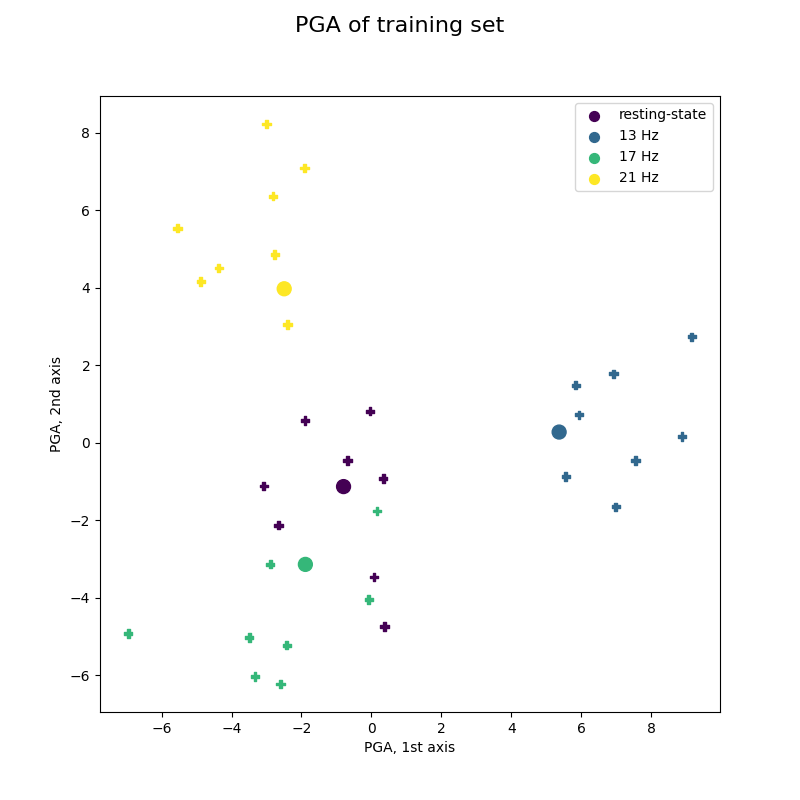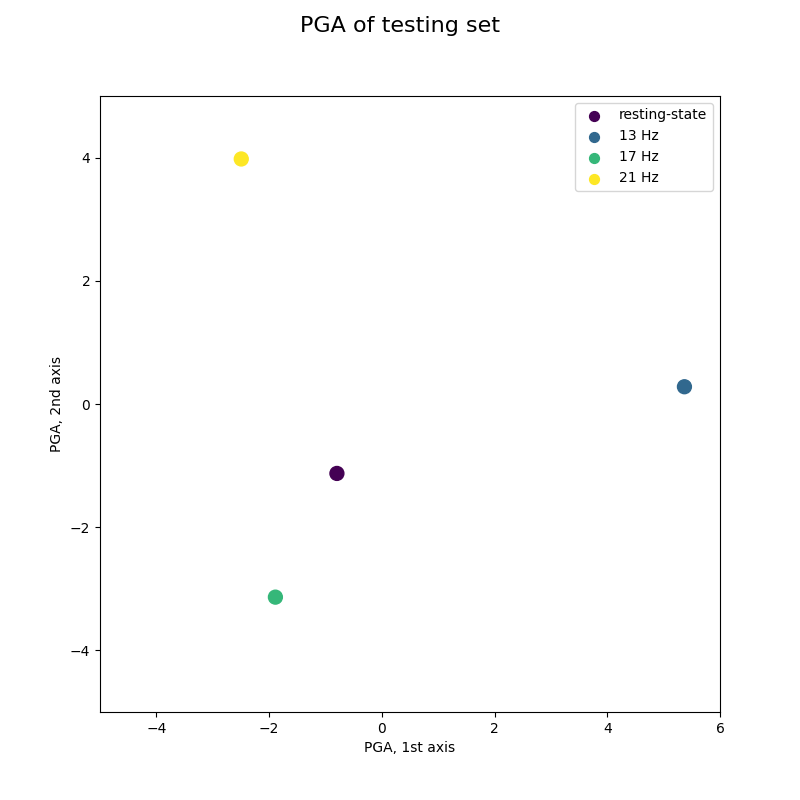# Visualization of SSVEP-based BCI Classification in Tangent Space¶

Project extended covariance matrices of SSVEP-based BCI in the tangent space, using principal geodesic analysis (PGA).

You should have a look to “Offline SSVEP-based BCI Multiclass Prediction” before this example.

```# Authors: Quentin Barthélemy, Emmanuel Kalunga and Sylvain Chevallier
#

import numpy as np
import matplotlib.pyplot as plt
from matplotlib.animation import FuncAnimation

from mne import find_events, Epochs, make_fixed_length_epochs
from mne.io import Raw
from sklearn.pipeline import make_pipeline
from sklearn.decomposition import PCA

from pyriemann.estimation import BlockCovariances
from pyriemann.classification import MDM
from pyriemann.tangentspace import TangentSpace
```
```clabel = ['resting-state', '13 Hz', '17 Hz', '21 Hz']
clist = plt.cm.viridis(np.array([0, 1, 2, 3])/3)
cmap = "viridis"

def plot_pga(ax, data, labels, centers):
sc = ax.scatter(data[:, 0], data[:, 1], c=labels, marker='P', cmap=cmap)
ax.scatter(
centers[:, 0], centers[:, 1], c=clist, marker='o', s=100, cmap=cmap
)
ax.set(xlabel='PGA, 1st axis', ylabel='PGA, 2nd axis')
for i in range(len(clabel)):
ax.scatter([], [], color=clist[i], marker='o', s=50, label=clabel[i])
ax.legend(loc='upper right')
return sc
```

## Load EEG and extract covariance matrices for SSVEP¶

```frequencies = [13, 17, 21]
freq_band = 0.1
events_id = {'13 Hz': 2, '17 Hz': 4, '21 Hz': 3, 'resting-state': 1}

duration = 2.5    # duration of epochs
interval = 0.25   # interval between successive epochs for online processing

# Subject 12: first 4 sessions for training, last session for test

# Training set
events = find_events(raw, shortest_event=0, verbose=False)
raw = raw.pick_types(eeg=True)
ch_count = len(raw.info['ch_names'])
raw_ext = extend_signal(raw, frequencies, freq_band)
epochs = Epochs(
raw_ext, events, events_id, tmin=2, tmax=5, baseline=None, verbose=False)
x_train = BlockCovariances(
estimator='lwf', block_size=ch_count).transform(epochs.get_data())
y_train = events[:, 2]

# Testing set
raw = raw.pick_types(eeg=True)
raw_ext = extend_signal(raw, frequencies, freq_band)
epochs = make_fixed_length_epochs(
raw_ext, duration=duration, overlap=duration - interval, verbose=False)
x_test = BlockCovariances(
estimator='lwf', block_size=ch_count).transform(epochs.get_data())
```
```Creating RawArray with float64 data, n_channels=24, n_times=92384
Range : 0 ... 92383 =      0.000 ...   360.871 secs
Using data from preloaded Raw for 32 events and 769 original time points ...

0%|                                              | 0.00/5.35M [00:00<?, ?B/s]
1%|▏                                     | 31.7k/5.35M [00:00<00:23, 227kB/s]
1%|▍                                     | 64.5k/5.35M [00:00<00:22, 231kB/s]
2%|▋                                     | 97.3k/5.35M [00:00<00:22, 232kB/s]
3%|█▏                                     | 163k/5.35M [00:00<00:15, 325kB/s]
7%|██▌                                    | 359k/5.35M [00:00<00:07, 712kB/s]
14%|█████▎                                | 753k/5.35M [00:00<00:03, 1.42MB/s]
25%|█████████▏                           | 1.33M/5.35M [00:01<00:01, 2.19MB/s]
29%|██████████▋                          | 1.54M/5.35M [00:01<00:01, 2.04MB/s]
50%|██████████████████▍                  | 2.67M/5.35M [00:01<00:00, 3.97MB/s]
69%|█████████████████████████▋           | 3.72M/5.35M [00:01<00:00, 5.06MB/s]
87%|████████████████████████████████▎    | 4.67M/5.35M [00:01<00:00, 5.58MB/s]
0%|                                              | 0.00/5.35M [00:00<?, ?B/s]
100%|█████████████████████████████████████| 5.35M/5.35M [00:00<00:00, 17.8GB/s]
Creating RawArray with float64 data, n_channels=24, n_times=148544
Range : 0 ... 148543 =      0.000 ...   580.246 secs
Using data from preloaded Raw for 2312 events and 640 original time points ...
```

## Classification with minimum distance to mean (MDM)¶

Classification for a 4-class SSVEP BCI, including resting-state class.

```print("Number of training trials: {}".format(len(x_train)))

mdm = MDM(metric=dict(mean='riemann', distance='riemann'))
mdm.fit(x_train, y_train)
```
```Number of training trials: 32

MDM(metric={'distance': 'riemann', 'mean': 'riemann'})
```

## Projection in tangent space with principal geodesic analysis (PGA)¶

Project covariance matrices from the Riemannian manifold into the Euclidean tangent space at the grand average, and apply a principal component analysis (PCA) to obtain an unsupervised dimension reduction 1.

```pga = make_pipeline(
TangentSpace(metric="riemann", tsupdate=False),
PCA(n_components=2)
)

ts_train = pga.fit_transform(x_train)
ts_means = pga.transform(np.array(mdm.covmeans_))
```

## Offline training of MDM visualized by PGA¶

These figures show the trajectory on the tangent space taken by covariance matrices during a 4-class SSVEP experiment, and how they are classified epoch by epoch.

This figure reproduces Fig 3(c) of reference 2, showing training trials of best subject.

```fig, ax = plt.subplots(figsize=(8, 8))
fig.suptitle('PGA of training set', fontsize=16)
plot_pga(ax, ts_train, y_train, ts_means)
plt.show()
```## Online classification by MDM visualized by PGA¶

This figure reproduces Fig 6 of reference 2, with an animation to imitate an online acquisition, processing and classification of EEG time-series.

Warning: 2 uses a curved based online classification, while a single trial classification is used here.

```# Prepare data for online classification
test_visu = 50     # nb of matrices to display simultaneously
colors, ts_visu = [], np.empty([0, 2])
alphas = np.linspace(0, 1, test_visu)

fig, ax = plt.subplots(figsize=(8, 8))
fig.suptitle('PGA of testing set', fontsize=16)
pl = plot_pga(ax, ts_visu, colors, ts_means)
pl.axes.set_xlim(-5, 6)
pl.axes.set_ylim(-5, 5)
``````(-5.0, 5.0)
```
```# Prepare animation for online classification
def online_classify(t):
global colors, ts_visu

# Online classification
y = mdm.predict(x_test[np.newaxis, t])
color = clist[int(y - 1)]
ts_test = pga.transform(x_test[np.newaxis, t])

# Update data
colors.append(color)
ts_visu = np.vstack((ts_visu, ts_test))
if len(ts_visu) > test_visu:
colors.pop(0)
ts_visu = ts_visu[1:]

# Update plot
pl.set_offsets(np.c_[ts_visu[:, 0], ts_visu[:, 1]])
pl.set_color(colors)
return pl

interval_display = 1.0  # can be changed for a slower display

visu = FuncAnimation(fig, online_classify,
frames=range(0, len(x_test)),
interval=interval_display, blit=False, repeat=False)
```
```# Plot online classification

# Plot complete visu: a dynamic display is required
plt.show()

# Plot only 10s, for animated documentation
try:
from IPython.display import HTML
except ImportError:
raise ImportError("Install IPython to plot animation in documentation")

plt.rcParams["animation.embed_limit"] = 10
HTML(visu.to_jshtml(fps=5, default_mode='loop'))
```
```Animation size has reached 10525072 bytes, exceeding the limit of 10485760.0. If you're sure you want a larger animation embedded, set the animation.embed_limit rc parameter to a larger value (in MB). This and further frames will be dropped.
```